•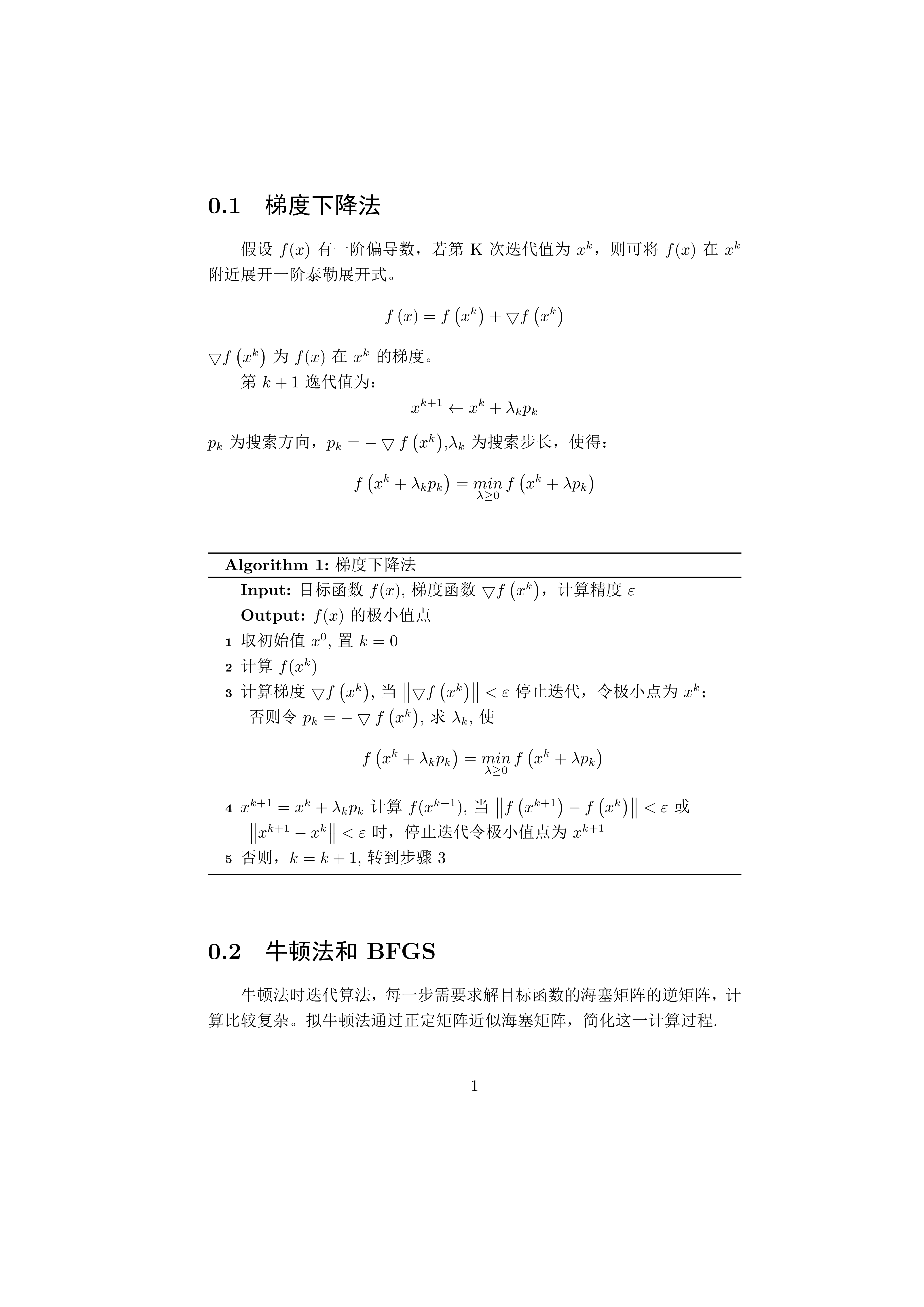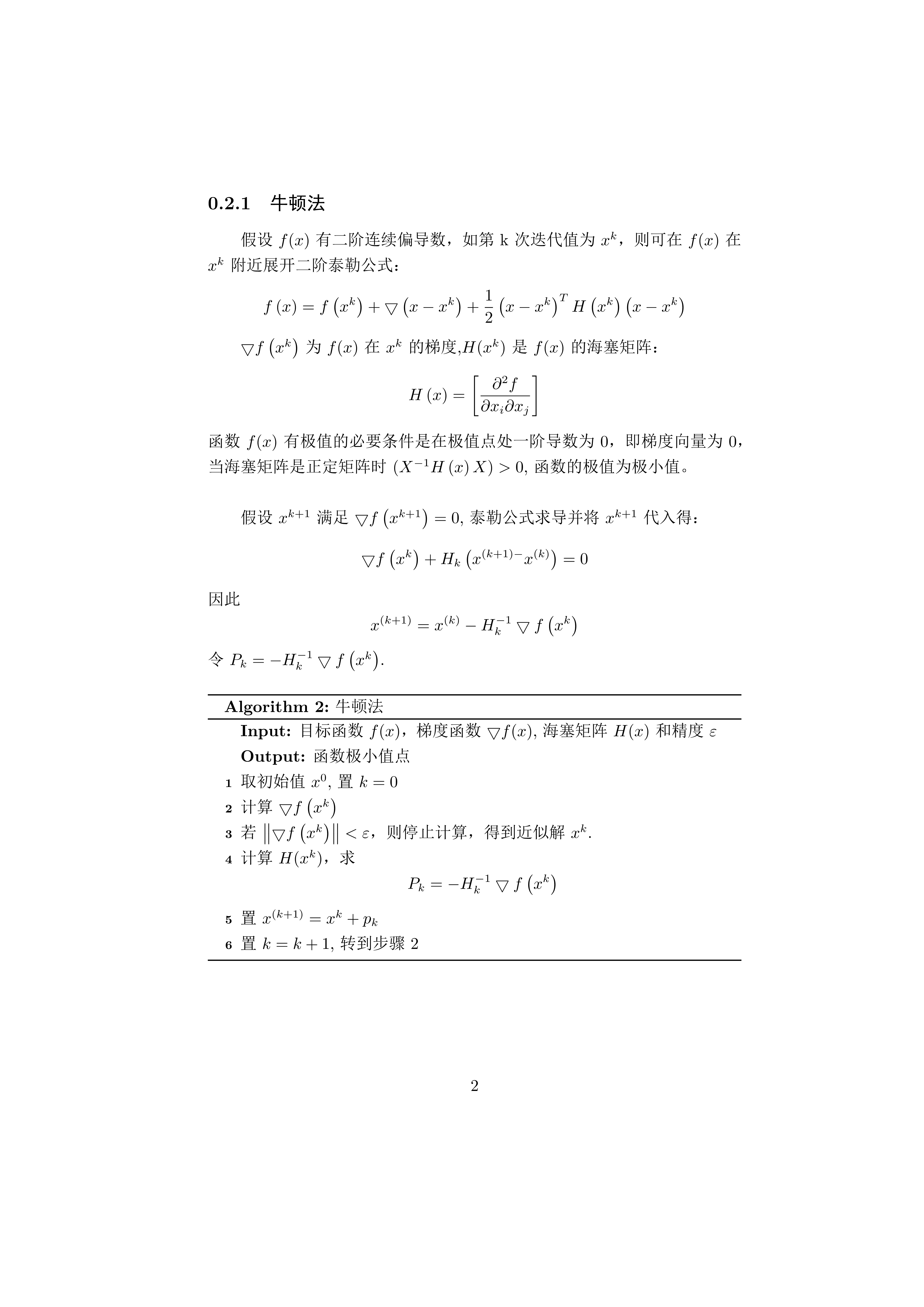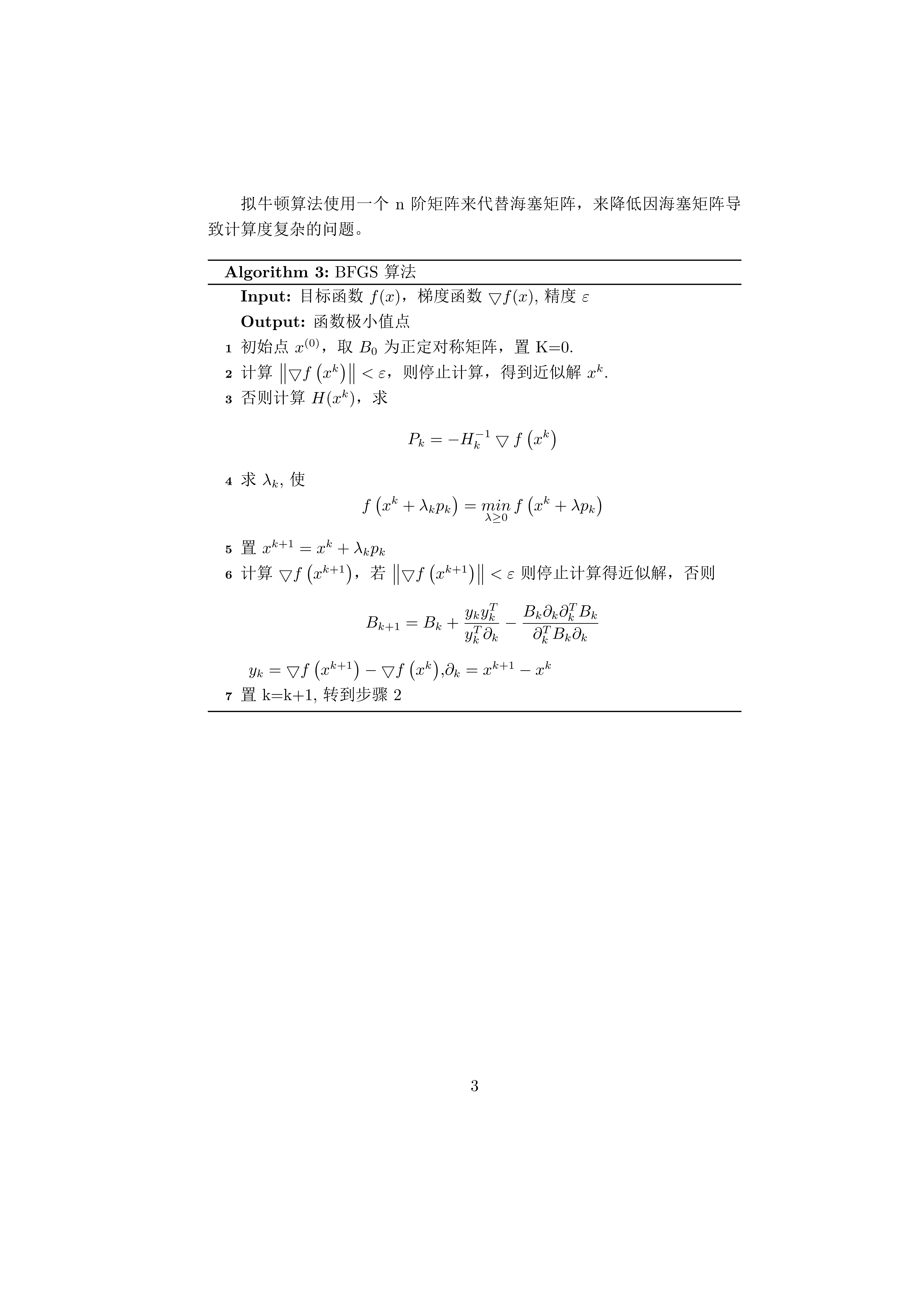展开全文梯度下降法 BFGS
• 约束最优化问题中，常常利用拉格朗日对偶性（Lagrange duality）将原始问题转换为对偶问题，通过解对偶问题而得到原始问题的解。该方法应用在许多统计学习方法中，例如最大熵模型和支持向量机。对于等式约束的优化...
在约束最优化问题中，常常利用拉格朗日对偶性（Lagrange duality）将原始问题转换为对偶问题，通过解对偶问题而得到原始问题的解。该方法应用在许多统计学习方法中，例如最大熵模型和支持向量机。
对于等式约束的优化问题，可以应用拉格朗日乘子法（Lagrange Multiplier)去求取最优值；如果含有不等式约束，可以应用KKT（Karush-Kuhn-Tucker）条件去求取。当然，这两个方法求得的结果只是必要条件，只有当是凸函数的情况下，才能保证是充分必要条件。
1. 拉格朗日乘子法和KKT条件
通常我们需要求解的最优化问题有如下几类：
无约束优化问题，可以写为:

minf(x);

min f(x); 有等式约束的优化问题，可以写为:

minf(x),s.t.hi(x)=0;i=1,...,n

\begin{align*}
min \, & f(x), \\
& s.t. h_i(x) = 0; i =1, ..., n \\
\end{align*}
有不等式约束的优化问题，可以写为：

minf(x),s.t.gi(x)<=0;i=1,...,nhj(x)=0;j=1,...,m

\begin{align*}
min \, & f(x), \\
& s.t. g_i(x) <= 0; i =1, ..., n \\
& \quad \; \; \, h_j(x) = 0; j =1, ..., m \\
\end{align*}

对于无约束优化问题，常常使用的方法就是Fermat定理，即使用求取

f(x)
$f(x)$ 的导数，然后令其为零，可以求得候选最优值，再在这些候选值中验证；如果是凸函数，可以保证是最优解。
对于有等式约束的优化问题，常常使用的方法就是拉格朗日乘子法，即把等式约束

hi(x)
$h_i(x)$ 用一个系数与

f(x)
$f(x)$ 写为一个式子，称为拉格朗日函数，而系数称为拉格朗日乘子。通过拉格朗日函数对各个变量求导，令其为零，可以求得候选值集合，然后验证求得最优值。
对于有不等式约束的优化问题，常常使用的方法就是KKT条件。同样地，我们把所有的等式、不等式约束与

f(x)
$f(x)$ 写为一个式子，也叫拉格朗日函数，系数也称拉格朗日乘子，通过一些条件，可以求出最优值的必要条件，这个条件称为KKT条件。
1.1 拉格朗日乘子法
对于等式约束，我们可以通过一个拉格朗日系数

a
$a$ 把等式约束和目标函数组合成为一个式子 L(a,x)=f(x)+a⋅h(x)$L(a, x) = f(x) + a\cdot h(x)$ , 这里把

a
$a$ 和 h(x)$h(x)$ 视为向量形式，

a
$a$ 是横向量，h(x)$h(x)$ 为列向量。
然后求取最优值，可以通过对

L(a,x)
$L(a,x)$ 对各个参数求导取零，联立等式进行求取。
1.2 KKT条件
对于含有不等式约束的优化问题，将其转化为对偶问题：

maxa,bminxL(a,b,x),s.t.ai≥0;i=1,...,n

\begin{align*}
\max\limits_{a,b} & \min\limits_x  L(a,b,x), \\
& s.t. a_i \geq 0; i =1, ..., n
\end{align*}

其中

L(a,b,x)
$L(a, b, x)$ 为由所有的不等式约束、等式约束和目标函数全部写成的一个式子

L(a,b,x)=f(x)+a⋅g(x)+b⋅h(x)
$L(a, b, x)= f(x) + a\cdot g(x)+b\cdot h(x)$，KKT条件是说原始问题最优值

x∗
$x^*$ 和对偶问题最优值

a∗,b∗
$a^*, b^*$ 必须满足以下条件：

∇xL(a∗,b∗,x∗)=0,∇aL(a∗,b∗,x∗)=0,∇bL(a∗,b∗,x∗)=0
$\nabla_x L(a^*, b^*, x^*)=0,\nabla_a L(a^*, b^*, x^*)=0,\nabla_b L(a^*, b^*, x^*)=0$；

a∗⋅gi(x∗)=0
$a^* \cdot g_i(x^*) = 0$;

gi(x∗)≤0
$g_i(x^*)\leq 0$;

a∗i≥0
$a_i^* \geq 0$,

hj(x)=0
$h_j(x) =0$.
当原始问题的解和对偶问题的解满足KKT条件，并且

f(x),gi(x)
$f(x),g_i(x)$ 是凸函数时，原始问题的解与对偶问题的解相等。
求取这几个等式之后就能得到候选最优值。其中第2个式子非常有趣，因为

gi(x∗)≤0
$g_i(x^*)\leq 0$ ，如果要满足这个等式，必须

a∗=0
$a^*=0$ 或者

gi(x∗)=0
$g_i(x^*)=0$. 这是SVM的很多重要性质的来源，如支持向量的概念。
2. 为什么拉格朗日乘子法和KKT条件能够得到最优值？
2.1 拉格朗日乘子法
设想我们想要最大化目标函数

f(x，y)
$f(x，y)$。可以投影在

x,y
$x,y$ 构成的平面（曲面）上，即成为等高线；假设我们的约束

g(x,y)=0
$g(x,y)=0$，在

x,y
$x,y$ 构成的平面或者曲面上是一条曲线，如下图。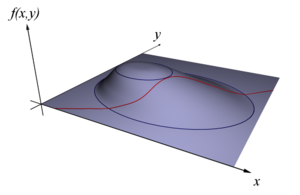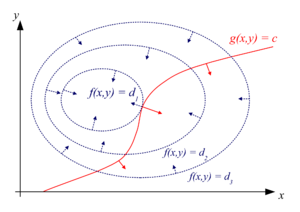图片来自维基百科
假设

g(x,y)
$g(x,y)$ 与等高线相交，交点就是同时满足等式约束条件和目标函数的可行域的值，但肯定不是最优值，因为相交意味着肯定还存在其它的等高线在该条等高线的内部或者外部，使得新的等高线与目标函数的交点的值更大或者更小，只有到等高线与目标函数的曲线相切的时候，才能取得最优值，即等高线和目标函数的曲线在该点的法向量必须有相同方向。
所以最优值必须满足：

∇f(x)=∇a⋅g(x)
$\nabla f(x) = \nabla{a\cdot g(x)}$，a是常数，表示左右两边同向，这个等式就是

L(a,x)
$L(a,x)$ 对参数求导的结果。
2.2 KKT条件
我们的目标问题是

minf(x)
$\min f(x)$。可以构造函数

L(a,b,x)
$L(a,b,x)$：

L(a,b,x)=f(x)+a⋅g(x)+b⋅h(x)，a≥0

L(a, b, x) = f(x) + a\cdot g(x) + b\cdot h(x)，a\geq 0

此时

f(x)
$f(x)$ 与

maxa,bL(a,b,x)
$\max\limits_{a,b} L(a,b,x)$ 是等价的。因为

h(x)=0,g(x)≤0,a⋅g(x)≤0
$h(x)=0, g(x) \leq 0, a\cdot g(x) \leq 0$，所以只有在

a⋅g(x)=0
$a\cdot g(x) = 0$ 的情况下

L(a,b,x)
$L(a,b,x)$ 才能取得最大值，这就是KKT的第二个条件。
因此我们的目标函数可以写为

minxmaxa,bL(a,b,x)
$\min\limits_x \max\limits_{a,b} L(a,b,x)$。如果用对偶表达式：

maxa,bminxL(a,b,x)
$\max\limits_{a,b} \min\limits_x L(a,b,x)$，由于我们的优化是满足强对偶的（强对偶就是说对偶式子的最优值是等于原问题的最优值的），所以在取得最优值

x∗
$x^*$ 的条件下，它满足

f(x∗)=maxa,bminxL(a,b,x)=minxmaxa,bL(a,b,x)=f(x∗)
$f(x^*) = \max\limits_{a,b} \min\limits_x L(a,b,x) = \min\limits_x \max\limits_{a,b} L(a,b,x) =f(x^*)$，我们来看看中间两个式子发生了什么事情：

f(x∗)====maxa,bminxL(a,b,x)maxa,bminx(f(x)+a⋅g(x)+b⋅h(x))maxa,b(f(x∗)+a⋅g(x∗)+b⋅h(x∗))f(x∗)

\begin{align*}
f(x^*) = &\max\limits_{a,b} \min\limits_x  L(a,b,x)  \\
= & \max\limits_{a,b} \min\limits_x \big( f(x) + a\cdot g(x) + b\cdot h(x) \big) \\
= & \max\limits_{a,b} \big( f(x^*)+a\cdot g(x^*)+b\cdot h(x^*)\big)  \\
=& f(x^*)
\end{align*}

可以看到上式本质上是说

minx(f(x)+a⋅g(x)+b⋅h(x))
$\min\limits_x \big( f(x) + a\cdot g(x) + b\cdot h(x)\big)$ 在

x∗
$x^*$ 取得了最小值，用fermat定理，即是说对于函数

f(x)+a⋅g(x)+b⋅h(x)
$f(x) + a\cdot g(x) + b\cdot h(x)$，求取导数要等于零，即

∇(f(x)+a⋅g(x)+b⋅h(x)=0)

\nabla \big( f(x) +  a\cdot g(x) +  b\cdot h(x) = 0\big)

这就是KKT条件中第一个条件：

∇xL(a,b,x)=0
$\nabla_x L(a, b, x)=0$。
所有上述说明，满足强对偶条件的优化问题的最优值都必须满足KKT条件。可以把KKT条件视为是拉格朗日乘子法的泛化。
参考资料：  1. 深入理解拉格朗日乘子法（Lagrange Multiplier) 和KKT条件  2. 李航，著. 统计学习方法[M]. 清华大学出版社，2012
展开全文• 数据理论上是呈现线性关系，但是实际数据往往不是满足线性关系，但大体...这个时候，我们最终希望得到的是唯一的线性关系，而不是一堆实验数据，所以产生了用一堆实验数据来确立理论上的线性关系的这样的问题。 ...
本文针对线性回归这一无约束最优化问题，深入分析最小二乘、梯度下降和牛顿法三种优化求解方法的优缺点，是对三种方法的原理学习总结。 无约束最优化问题，是指在一个给定的集合S上泛函J(θ)的极小化或极大化问题。
1.应用场景引入
数据间往往大体趋势呈现出线性关系，但并不完全满足。我们希望得到唯一的线性关系，表示输入数据到输出数据之间映射的函数，使其很好的拟合已知函数并预测未知数据，即线性回归问题。
最小二乘法是一种确立数据间最好的映射函数的优化技术，梯度下降和牛顿法是两种可根据这种优化技术中的评估准则求解最优映射函数的具体方法。
最小二乘法：以偏差的最小平方和为评估准则，求解最佳映射函数回归系数的解析解，结果为全局最优； 梯度下降和牛顿法：两种通过迭代更新来逐步进行的参数优化方法，假设条件更为广泛（无约束）的，结果一般为局部最优；
2.从最大似然解释最小二乘
最大似然估计是一种“模型已定，参数未知”的方法。利用已知的样本的结果，在使用某个模型的基础上，反推最有可能导致这样结果的模型参数值。 根据高斯-马尔科夫定理，在线性回归模型中，如果误差满足零均值、同方差且互不相关，则回归系数的最佳线性无偏估计(BLUE, Best Linear unbiased estimator)就是普通最小二乘法估计。特别地，当随机噪声服从正态分布时，最小二乘与最大似然等价。 求解最大似然估计的一般过程为：
写出似然函数（生成已知样本的联合概率）；如果无法直接求导的话，对似然函数取对数；求导数 ；求解模型中参数的最优值。
线性回归模型的似然函数为：假设随机误差 ε是独立同分布的，服从数学期望为μ、方差为σ^2 的正态分布（中心极限定理）。误差 ε的概率密度函数为：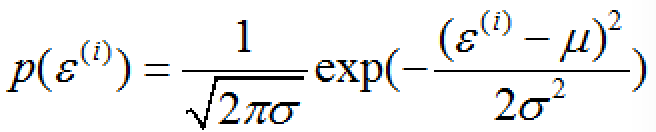y、x取值的概率密度函数：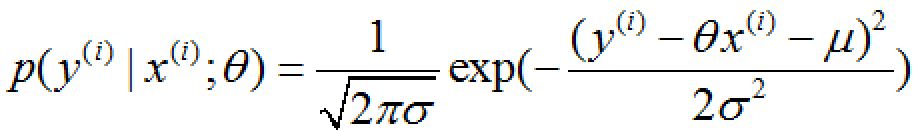联合概率无法获取的时候，概率密度积最大便最大似然。由此得到线性回归模型参数θ的似然估计：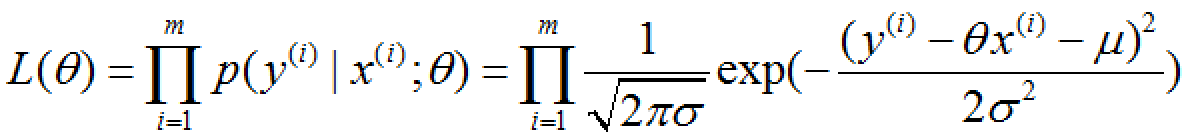对似然估计取对数：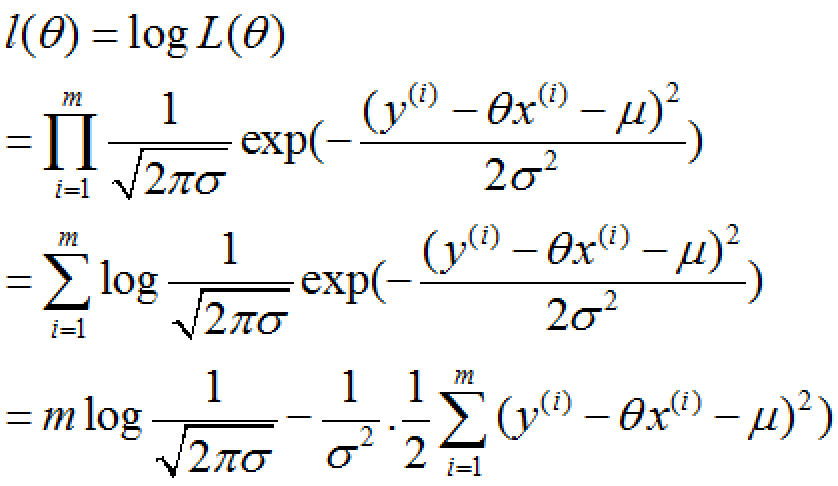要使 l(θ) 最大，则上式减号右边的式子最小，即下式最小。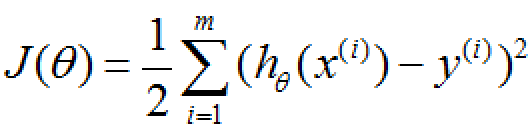与最小二乘的评价准则偏差的最小平方和相同，由此可解释当随机噪声服从正态分布时，最小二乘与最大似然等价。同时证明了最小二乘的合理性。
最小二乘法：即观测值与实际数据误差平方和最小，其没有假设，几何意义上就是距离最小。 最大似然估计：估计参数可能值，使样本发生概率最大。
3.最小二乘求解线性回归系数
设一元线性回归模型为y=ax+b。 根据最小二乘的评价准则，使得axi+b与yi的偏差平方和最小的参数a、b确定了源数据（xi，yi）的最优回归直线。即确定二元函数：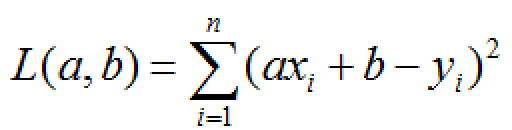的最小值。若L(a,b)的极值就是最小值即存在最优回归直线，那么Hessian矩阵：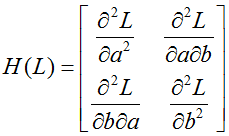就必须是正定的。求二元函数L的二阶偏导：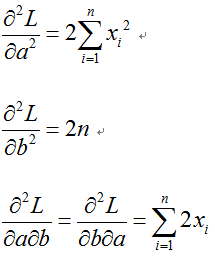则H(L)的行列式：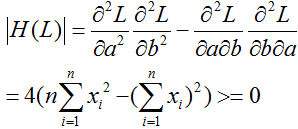又因为1阶顺序主子式大于0，所以H(L)正定。最小值存在，最优回归直线存在。 所以L(a,b)的驻点就是极小值点。利用克拉默法则，首先令L(a,b)的一阶偏导：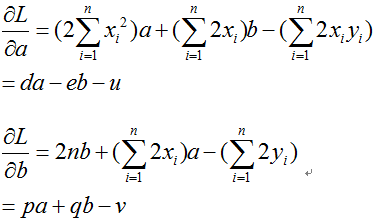等于0，得到系数行列式D：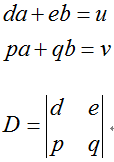D不等于0，线性方程组有唯一解：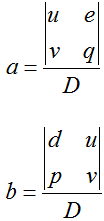于是通过最小二乘法得到最优归回直线：y=ax+b。
3.梯度下降法
3.1梯度
在解决线性回归问题时，针对最小二乘法中的偏差平方和评估准则，可以通过梯度下降法来一步步的迭代求解，得到最小平方和的映射函数参数值。
假设L(θ)为偏差平方和函数，其中θ为要求解的映射函数参数。梯度下降法是一种 迭代方法:选取初值θ0，不断迭代，更新θ的值，进行L(θ)的极小化。
迭代公式：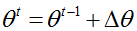泰勒函数是一个用函数在某点的信息描述其附近取值的公式，具有局部有效性。将L(θt)在L(θt-1)处进行一阶泰勒展开：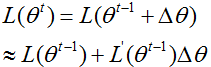要使得：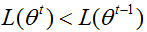可取：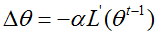则：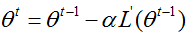这里∂是步长，可通过line search确定，但一般直接赋一个小的数。
梯度下降不一定能够找到全局的最优解，有可能是一个局部最优解。当然，如果损失函数是凸函数，梯度下降法得到的解就一定是全局最优解。
3.2批量梯度下降法
批量梯度下降法，是梯度下降法最常用的形式，具体做法也就是在更新参数时使用所有的样本来进行更新，迭代公式为：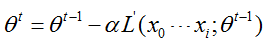3.3随机梯度下降法
随机梯度下降法，其实和批量梯度下降法原理类似，区别在与求梯度时没有用所有的m个样本的数据，而是仅仅选取一个样本来求梯度。迭代公式为：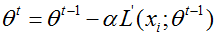3.4小批量梯度下降法
小批量梯度下降法是批量梯度下降法和随机梯度下降法的折衷，也就是可以选取m+1个样本来迭代，即若干个样本的平均梯度作为更新方向，根据样本的数据，可以调整选取样本的数量。迭代公式为：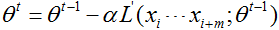对于训练速度来说，随机梯度下降法由于每次仅仅采用一个样本来迭代，训练速度很快，而批量梯度下降法在样本量很大的时候，训练速度不能让人满意。对于准确度来说，随机梯度下降法用于仅仅用一个样本决定梯度方向，导致解很有可能不是最优。对于收敛速度来说，由于随机梯度下降法一次迭代一个样本，导致迭代方向变化很大，不能很快的收敛到局部最优解。所以说小批量梯度下降法是一种性能折中的设计。
4.牛顿法
将L(θt)在L(θt-1)处进行二阶泰勒展开：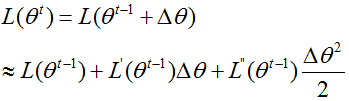为了简化分析过程，假设参数是标量(即θ只有一维)，则可将一阶和二阶导数分别记为g和h：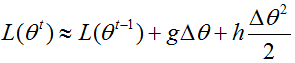要使得L(θt)极小，即让右侧后两项极小，可令：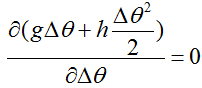求得：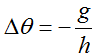故：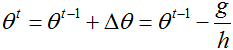参数θ推广到向量形式，迭代公式为：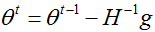这里H是海森矩阵(Hesse)。 牛顿法需要求Hesse矩阵的逆,容易出现数值不稳定的情况。拟牛顿法不是直接去求Hesse矩阵,而是通过梯度函数g和两个X点的位移来构造与Hesse相似的正定矩阵来代替,减少运算的复杂度.
5.区别与优缺点总结
最小二乘法和梯度下降、牛顿法相比：
梯度下降与牛顿法是迭代求解，最小二乘法是计算解析解。  迭代求解需要选择步长，快迭代到最小点的时候收敛速度变慢，并且对初始点的选择极为敏感，而且往往只能找到局部最小。  如果样本量不算很大，且存在解析解，最小二乘法比起梯度下降与牛顿法要有优势，计算速度很快。但是如果样本量很大，用最小二乘法由于需要求一个超级大的逆矩阵，这时就很难或者很慢才能求解解析解了，使用迭代的梯度下降与牛顿法比较有优势。
梯度下降法和牛顿法相比：
两者都是迭代求解，不过梯度下降法是梯度求解，而牛顿法是用二阶的海森矩阵的逆矩阵求解。相对而言，使用牛顿法收敛更快。但是每次迭代的时间比梯度下降法长。
展开全文机器学习 梯度下降 最小二乘
• 不等式约束优化问题求解 与前文讨论的只含等式约束优化问题求解类似，含不等式约束优化问题同样可以用拉格朗日乘子法进行求解 对于一般形式的优化问题： minimizef(x)subject&nbsp;toh(x)=0g(x)≤0...
不等式约束的优化问题求解
与前文讨论的只含等式约束的优化问题求解类似，含不等式约束的优化问题同样可以用拉格朗日乘子法进行求解  对于一般形式的优化问题：

minimizef(x)subject toh(x)=0g(x)≤0

m

i

n

i

m

i

z

e

f

(

x

)

s

u

b

j

e

c

t

t

o

h

(

x

)

=

0

g

(

x

)

≤

0

其中，

f:Rn→R,h:Rn→Rm,m≤n,g:Rn→Rp

f

:

R

n

→

R

,

h

:

R

n

→

R

m

,

m

≤

n

,

g

:

R

n

→

R

p

$f:R^{n}\to R,h:R^{n}\to R^{m},m\le n,g:R^{n}\to R^{p}$
引入下面两个定义：

定义1：对于一个不等式约束

gj(x)≤0

g

j

(

x

)

≤

0

$g_{j}(x)\le 0$，如果在

x∗

x

∗

$x^{*}$处

gj(x∗)=0

g

j

(

x

∗

)

=

0

$g_{j}(x^{*})=0$，那么称该不等式约束是

x∗

x

∗

$x^{*}$处的起作用约束；如果在

x∗

x

∗

$x^{*}$处

gj(x∗)<0

g

j

(

x

∗

)

<

0

$g_{j}(x^{*})<0$，那么称该约束是

x∗

x

∗

$x^{*}$处的不起作用约束。按照惯例，总是把等式约束

hi(x)

h

i

(

x

)

$h_{i}(x)$当作起作用的约束
定义2：设

x∗

x

∗

$x^{*}$满足

h(x∗)=0,g(x∗)≤0

h

(

x

∗

)

=

0

,

g

(

x

∗

)

≤

0

$h(x^{*})=0,g(x^{*})\le 0$，设

J(x∗)

J

(

x

∗

)

$J(x^{*})$为起作用不等式约束的下标集：

J(x∗)≜{j:gj(x∗)=0}

J

(

x

∗

)

≜

{

j

:

g

j

(

x

∗

)

=

0

}

J(x^{*})\triangleq \{j:g_{j}(x^{*})=0\}

如果向量

∇hi(x∗),∇gj(x∗),1≤i≤m,j∈J(x∗)

∇

h

i

(

x

∗

)

,

∇

g

j

(

x

∗

)

,

1

≤

i

≤

m

,

j

∈

J

(

x

∗

)

\nabla h_{i}(x^{*}),\nabla g_{j}(x^{*}),1\le i\le m,j\in J(x^{*})

是线性无关的，那么称

x∗

x

∗

$x^{*}$是一个正则点

下面介绍某个点是局部极小点所满足的一阶必要条件，即KKT条件。  KKT条件：设

f,h,g∈C1

f

,

h

,

g

∈

C

1

$f,h,g\in C^{1}$，设

x∗

x

∗

$x^{*}$是问题

h(x)=0,g(x)≤0

h

(

x

)

=

0

,

g

(

x

)

≤

0

$h(x)=0,g(x)\le 0$的一个正则点和局部极小点，那么必然存在

λ∗∈Rm

λ

∗

∈

R

m

$\lambda^{*}\in R^{m}$和

μ∗∈Rp

μ

∗

∈

R

p

$\mu^{*}\in R^{p}$，使得以下条件成立：

μ∗≥0Df(x∗)+λ∗TDh(x∗)+μ∗TDg(x∗)=0Tμ∗Tg(x∗)=0h(x∗)=0g(x∗)≤0

μ

∗

≥

0

D

f

(

x

∗

)

+

λ

∗

T

D

h

(

x

∗

)

+

μ

∗

T

D

g

(

x

∗

)

=

0

T

μ

∗

T

g

(

x

∗

)

=

0

h

(

x

∗

)

=

0

g

(

x

∗

)

≤

0

\mu^{*}\ge0\\
Df(x^{*})+\lambda^{*T}Dh(x^{*})+\mu^{*T}Dg(x^{*})=0^{T}\\
\mu^{*T}g(x^{*})=0\\
h(x^{*})=0\\
g(x^{*})\le0

那么在求解不等式约束的最优化问题的时候，可以搜索满足KKT条件的点，并将这些点作为极小点的候选对象。

二阶充分必要条件
除了一阶的KKT条件之外，求解这类问题还有二阶的充分必要条件。
二阶必要条件：在上述的问题中若

x∗

x

∗

$x^{*}$是极小点且

f,h,g∈C2

f

,

h

,

g

∈

C

2

$f,h,g\in C^{2}$。假设

x∗

x

∗

$x^{*}$是正则点，那么存在

λ∗∈Rm

λ

∗

∈

R

m

$\lambda^{*}\in R^{m}$和

μ∗∈Rp

μ

∗

∈

R

p

$\mu^{*}\in R^{p}$使得

μ∗≥0,Df(x∗)+λ∗TDh(x∗)+μ∗TDg(x∗)=0T,μ∗Tg(x∗)=0

μ

∗

≥

0

,

D

f

(

x

∗

)

+

λ

∗

T

D

h

(

x

∗

)

+

μ

∗

T

D

g

(

x

∗

)

=

0

T

,

μ

∗

T

g

(

x

∗

)

=

0

$\mu^{*}\ge 0,Df(x^{*})+\lambda^{*T}Dh(x^{*})+\mu^{*T}Dg(x^{*})=0^{T},\mu^{*T}g(x^{*})=0$对于所有

y∈T(x∗)

y

∈

T

(

x

∗

)

$y\in T(x^{*})$，都有

yTL(x∗,λ∗,μ∗)y≥0

y

T

L

(

x

∗

,

λ

∗

,

μ

∗

)

y

≥

0

$y^{T}L(x^{*},\lambda^{*},\mu^{*})y\ge0$成立
二阶充分条件：假定

f,h,g∈C2

f

,

h

,

g

∈

C

2

$f,h,g\in C^{2}$，

x∗∈Rn

x

∗

∈

R

n

$x^{*}\in R^{n}$是一个可行点，存在向量

λ∗∈Rm

λ

∗

∈

R

m

$\lambda^{*}\in R^{m}$和

μ∗∈Rp

μ

∗

∈

R

p

$\mu^{*}\in R^{p}$使得

μ∗≥0,Df(x∗)+λ∗TDh(x∗)+μ∗TDg(x∗)=0T,μ∗Tg(x∗)=0

μ

∗

≥

0

,

D

f

(

x

∗

)

+

λ

∗

T

D

h

(

x

∗

)

+

μ

∗

T

D

g

(

x

∗

)

=

0

T

,

μ

∗

T

g

(

x

∗

)

=

0

$\mu^{*}\ge 0,Df(x^{*})+\lambda^{*T}Dh(x^{*})+\mu^{*T}Dg(x^{*})=0^{T},\mu^{*T}g(x^{*})=0$对于所有

y∈T˜(x∗,μ∗),y≠0

y

∈

T

~

(

x

∗

,

μ

∗

)

,

y

≠

0

$y\in \widetilde{T}(x^{*},\mu^{*}),y\neq0$，都有

yTL(x∗,λ∗,μ∗)y>0

y

T

L

(

x

∗

,

λ

∗

,

μ

∗

)

y

>

0

$y^{T}L(x^{*},\lambda^{*},\mu^{*})y>0$成立
那么

x∗

x

∗

$x^{*}$是优化问题

h(x)=0,g(x)≤0

h

(

x

)

=

0

,

g

(

x

)

≤

0

$h(x)=0,g(x)\le 0$的严格局部极小点
展开全文• ## MATLAB有约束最优化问题的求解

万次阅读 多人点赞 2019-11-09 19:47:38
约束最优化问题的一般描述为： 其中x = [x1，x2，…，xn]T，该数学表示的含义亦即求取一组x，使得目标函数f（x）为最小，且满足约束条件G(x) ≤0。记号s.t.是英文subject to的缩写，表示x要满足后面的约束条件...
• 1. 问题描述最优化问题的一般形式如下所示： 对于f:D⊆Rn→R1f:D\subseteq R^n \rightarrow R^1，求解 minx∈Ωf(x)s.t.{s(x)⩾0h(x)=0 \min_{x\in\Omega} f(x) \qquad s.t. \left\{ \begin{aligned} s(x)\...最速下降法
• 基于Matlab工具的遗传算法求解约束最优化问题
• 基于Matlab工具的遗传算法求解约束最优化问题.pdf
• 首先感谢《利用Python求解约束最优化问题》让我不用自己重0开始敲代码了！不过就是效率有点慢了，还在解决中，至少问题有解了~ =================== 问题描述 ======================= 昨天项目例会上产品提了一...
• 使用scipy中的minimize函数求解最优化问题的形式如下： 其中xxx是一个向量，gi(x)g_{i}(x)gi​(x)是非等式约束，hj(x)h_{j}(x)hj​(x)是等式约束。 例7.1中的优化问题如下： 刚好能够带到式子里面，下面是代码： ...scipy python
• MATLAB在遗传算法中的应用，以求解约束最优化问题matlab
• 最后总结一句话，牛顿法虽然收敛速度快，但是对a点的选取有比较苛刻的要求，因此要使用牛顿法，必须对问题做一些预处理，比如先用速下降法求出一个与极值点相近的点，然后再从这个相近的点开始用牛顿法最终求出...泰勒公式 数学规划
• 基于Matlab工具的遗传算法求解约束最优化问题_刘鲭洁.pdf
• 基于Matlab工具的遗传算法求解约束最优化问题遗传算法
• 今天小编就为大家分享一篇使用Python求解约束最优化问题详解，具有很好的参考价值，希望对大家有所帮助。一起跟随小编过来看看吧
• #导入sympy包，用于求导，方程组求解等等 from sympy import * #设置变量 x1 = symbols("x1") x2 = symbols("x2") alpha = symbols("alpha") beta = symbols("beta") #构造拉格朗日等式 L = 10 - x1*x1 - x2*x2...Python
• 前言：由于本科学习的数理知识较为基础，没有涉及到优化问题相关知识，在遇到优化问题时，满脸问号这是个啥子嘞？相信很多小伙伴也有和我一样的感受。在网上一顿神搜索，又查阅了相关的书籍，终于有了一点感悟。由于...算法
• 我们要求解的最优化问题的形式如下： min f(x)s.t.gi(x)&gt;0,i=1,...,mhj(x)=0,j=1,...,n \begin{aligned} min \ f(x) \\ s.t.\quad g_i(x)&amp; \gt 0, i = 1,...,m\\ \quad h_j(x)&amp; = ...python scipy
• ## 约束优化问题的罚函数求解方法

万次阅读 多人点赞 2018-06-03 20:01:25
惩罚函数法是求解约束最优化问题的一种算法。 惩罚函数法的要旨是将一个有约束最优化问题转化为一系列的无约束问题；这些无约束问题由原问题及罚函数，再加上惩罚因子组成；而且，这些无约束问题的解会收敛...罚函数 大数据
• 以SVM优化问题为例，其回归函数（分类平面）为： 优化问题的目标函数为： SVM中的分类问题和预测问题的区别在于约束条件的不同。 在分类问题中，约束条件为：；而在预测问题中，约束条件为（以Vapnik 不敏感...算法
• Lingo是一款求解最优化问题的软件，可以用于求解非线性规划，也可以用于一些线性和非线性方程组的求解等，功能十分强大，是求解优化模型的最佳选择。 最优化问题 首先介绍一下什么是最优化问题最优化问题，即在...数学建模
• 请问，如果matlab 优化当中， 目标函数 和 约束条件都很复杂，没有办法直接用x 表示出来，该怎么处理？ 而且，对于要求出的x， 我有多个目标函数，这又该如何处理？例如：图像1， 图像2 ，图像3， 图像4，图像5 。。...
• 在无约束最优化问题的基础上，我们可以进一步来求解约束最优化问题约束最优化问题的一般形式为： minf(x)s.t.gi(x)≥0,i=1,...,m \begin{aligned} &\min f(x) \\ &s.t. \quad g_i(x)\ge0, i=1,...,m \end{...优化 Frank Wolfe
• 老外编写的牛顿截断法求解带边界约束的最优规划程序...